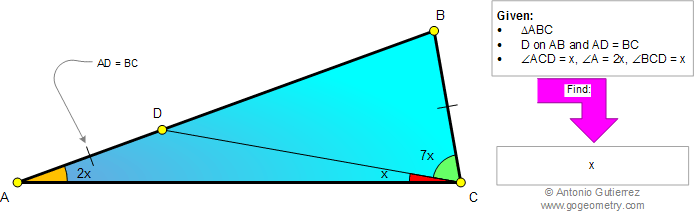# Online Geometry Problem 882: Triangle, Angle x, 2x, 7x, Cevian, Congruence, Auxiliary Lines. Level: High School, Honors Geometry, College, Mathematics Education

 In a triangle ABC, D is on AB so that AD = BC, angle ACD = x, angle A = 2x, and angle BCD = 7x. Find x.Geometry problem solving is one of the most challenging skills for students to learn. When a problem requires auxiliary construction, the difficulty of the problem increases drastically, perhaps because deciding which construction to make is an ill-structured problem. By “construction,” we mean adding geometric figures (points, lines, planes) to a problem figure that wasn’t mentioned as "given."
 Home | Search | Geometry | Problems | All Problems | Open Problems | Visual Index | 10 Problems | Problems Art Gallery |  Art | 881-890 | Triangle | Double Angle | Congruence | Angle | Solution / comment | By Antonio Gutierrez